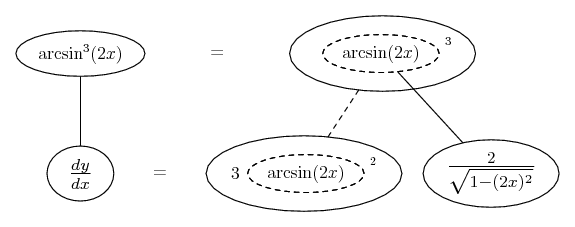# Thread: Inverse trigonometric functions

1. ## Inverse trigonometric functions

Differentiate with respect to x:

y = (arcsin 2x)^3

I obtained:

dy/dx = 6(arcsin 2x)^2

although, according to the answer, what I gave was either du/dx or dy/du. Can someone please point out how to get du/dx and dy/du in this case. I dont know how to apply the standard inverse trig function for sin^-1 here.

2. Just in case a picture helps...... where... is the chain rule. Straight continuous lines differentiate downwards (integrate up) with respect to x, and the straight dashed line similarly but with respect to the dashed balloon expression (the inner function of the composite which is subject to the chain rule).

Note: other brands of chain rule are available! My dashed balloon is really u.

I've skirted the issue of the chain rule for differentiating arcsin(2x). You could draw a similar pic with 2x the dashed balloon expression.

__________________________________________

Don't integrate - balloontegrate!

Balloon Calculus; standard integrals, derivatives and methods

Balloon Calculus Drawing with LaTeX and Asymptote!

3. ##$\displaystyle \forall x\in ]-1,1[,(\arcsin(x))'=\frac{1}{\sqrt{1-x^2}}$.
functions, inverse, trigonometric